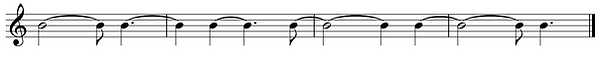top of page

CREATIVE SUBDIVISION

Let start with his Creative subdivision system. A Subdivision is dividing a rhythmic unit into a smaller unit. Ronan indicates that we tend to use always the same type of subdivision in our compositions or improvisation. With his Creative Subdivision system, he found a way to divide a unit into smaller units. This results in subdivisions that we would not normally think of so quickly.

He sees every rhythm as either 3 or 2 or a combination of 3 and/or 2. A dotted quarter note for example is a 3 (3 eight notes), and a half note is a 2 (2 quarter notes). If we combine this, for example for a group of 5, we have either 2+3 or 3+2. And a group of 7 could be 3+2+2 or 2+3+3 or 3+2+3. Using this principal is very easy to come up with some interesting rhythmic combinations over a basic 4/4.

Example: 3+2+2+2+3+3+2+3+3+2+2+2+3Example: grouping with a combination of 3 & 2: 5+5+5+7+7+3.In this example we have 8th notes in groups of 5 and 7 (with one group of 3 notes to form the cycle of 4 bar).

If you put notes to these types of rhythms, you can come up with very original rhythmic melodies. This method is open to almost infinite variation. By practicing this principal, you can begin opening up your rhythmically playing and create fresher melodic shapes as an improviser and composer.

bottom of page